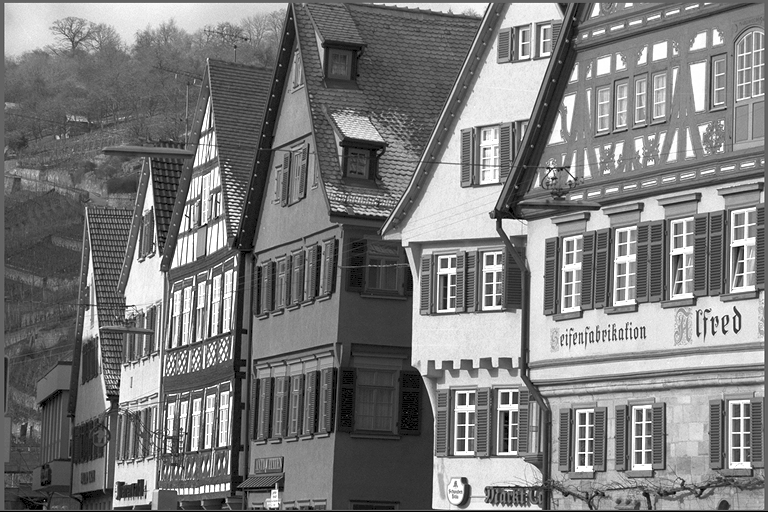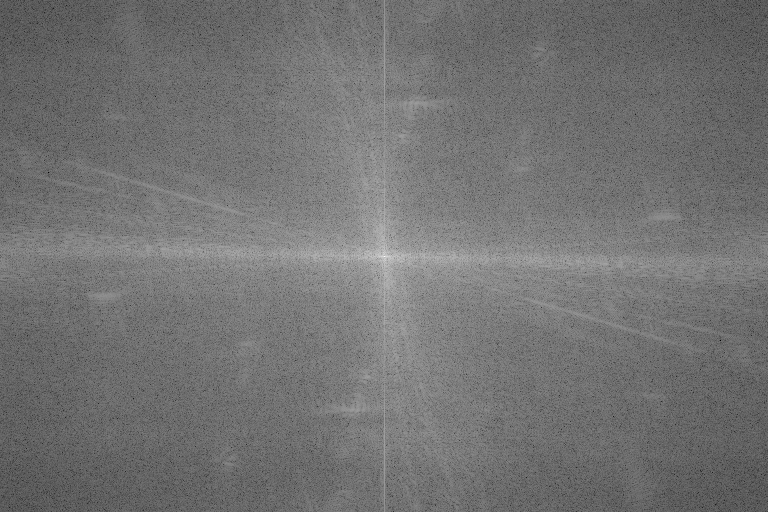FFT

# Overview

FFT implements the Fourier Transform applied to 2D images, supporting real- and complex-valued inputs. It returns the image representation in the frequency domain. It is useful for content analysis based on signal frequencies, and enables image operations that are more efficiently performed on the frequency domain, among other uses.

Input in space domain Magnitude spectrum# Implementation

The FFT - Fast Fourier Transform is a divide-and-conquer algorithm for efficiently computing discrete Fourier transforms of complex or real-valued data sets in $$O(n \log n)$$. It is one of the most important and widely used numerical algorithms in computational physics and general signal processing.

The Discrete Fourier Transform (DFT) generally maps a complex-valued vector $$x_k$$ on the space domain into its frequency domain representation given by:

$I'[u,v] = \sum^{M-1}_{m=0} \sum^{N-1}_{n=0} I[m,n] e^{-2\pi i (\frac{um}{M}+\frac{vn}{N})}$

Where:

• $$I$$ is the input image in spatial domain
• $$I'$$ is its frequency domain representation
• $$M\times N$$ is input's dimensions

Note that the $$I'$$ is left denormalized as the spectrum is eventually normalized in subsequent stages of the pipeline (if at all).

Depending on image dimension, different techniques are employed for best performance:

• CPU backend
• Fast paths when $$M$$ or $$N$$ can be factored into $$2^a \times 3^b \times 5^c$$
• CUDA backend
• Fast paths when $$M$$ or $$N$$ can be factored into $$2^a \times 3^b \times 5^c \times 7^d$$

In general the smaller the prime factor is, the better the performance, i.e., powers of two are fastest.

FFT supports the following transform types:

• complex-to-complex, C2C
• real-to-complex, R2C.

## Data Layout

Data layout depends strictly on the transform type. In case of general C2C transform, both input and output data shall be of type VPI_IMAGE_FORMAT_2F32 and have the same size. In R2C mode, each input image row $$(x_1,x_2,\dots,x_N)$$ with type VPI_IMAGE_FORMAT_F32 results in an output row $$(X_1,X_2,\dots,X_{\lfloor\frac{N}{2}\rfloor+1})$$ of non-redundant complex elements with type VPI_IMAGE_FORMAT_2F32. In both cases, input and output image heights are the same.

FFT typeinputoutput
typesizetypesize
C2CVPI_IMAGE_FORMAT_2F32$$W \times H$$VPI_IMAGE_FORMAT_2F32$$W \times H$$
R2CVPI_IMAGE_FORMAT_F32$$W \times H$$VPI_IMAGE_FORMAT_2F32$$\big( \lfloor\frac{W}{2}\rfloor+1 \big) \times H$$

For real inputs, the output contains only the non-redundant elements, thus only the left half of spectrum returned. In order to retrieve the whole Hermitian (symmetric-conjugate) output, the right half must be completed so that the following conditions are met:

\begin{align*} F_{\textbf{re}}(u,v) &= F_{\textbf{re}}(-u,-v) \\ F_{\textbf{re}}(-u,v) &= F_{\textbf{re}}(u,-v) \\ F_{\textbf{im}}(u,v) &= -F_{\textbf{im}}(-u,-v) \\ F_{\textbf{im}}(-u,v) &= -F_{\textbf{im}}(u,-v) \end{align*}

The following diagram shows how this is accomplished:

Completing the output spectrum

The zero-th element in spectrum output represents the DC (0 Hz) component. Usually for visualization it's preferred that DC is at the center. One way to accomplish this is to shift the 4 quadrants around as shown in the diagram below:

 $$Q_0$$ $$Q_1$$ $$Q_2$$ $$Q_3$$

$$\rightarrow$$

 $$Q_3$$ $$Q_2$$ $$Q_1$$ $$Q_0$$

# C API functions

For list of limitations, constraints and backends that implements the algorithm, consult reference documentation of the following functions:

Function Description
vpiCreateFFT Creates payload for direct Fast Fourier Transform algorithm.
vpiSubmitFFT Runs the direct Fast Fourier Transform on single image.

# Usage

Language:
1. Import VPI module
import vpi
2. Optionally convert the input to 32-bit floating point and apply the FFT operation. The resulting image will have complex components, represented by vpi.Format._2F32 format. All operations will be executed by the CUDA backend.
with vpi.Backend.CUDA:
output = input.convert(vpi.Format.F32) \
.fft()
3. (optional) Convert the complex frequency data into magnitude ready to get displayed.
1. Copy the output to a numpy array and convert it to np.complex64
hfreq = output.cpu().view(dtype=np.complex64).squeeze(2)
2. Fills the right half of the complex data with the missing values. The left half is copied directly from VPI's output. The result is a full Hermitian matrix.
if input.width%2==0:
else:
freq[:,hfreq.shape:] = np.conj(freq[:,hfreq.shape:])
freq[1:,hfreq.shape:] = freq[1:,hfreq.shape:][::-1]
3. Convert the complex frequencies into normalized log-magnitude
lmag = np.log(1+np.absolute(freq))
4. Shift the spectrum so that DC is at center
spectrum = np.fft.fftshift(lmag)
1. Initialization phase
1. Include the header that defines the image FFT function and the needed image format conversion functionality.
#include <vpi/algo/FFT.h>
Declares functions that handle image format conversion.
Declares functions that implement the Fast Fourier Transform algorithm and its inverse.
2. Define the input image object.
VPIImage input = /*...*/;
struct VPIImageImpl * VPIImage
A handle to an image.
Definition: Types.h:256
3. Create the output image that will hold the spectrum. Since input is real, only non-redundant values will be calculated and output width is $$\lfloor \frac{w}{2} \rfloor+1$$.
int width, height;
vpiImageGetSize(input, &width, &height);
VPIImage spectrum;
vpiImageCreate(width / 2 + 1, height, VPI_IMAGE_FORMAT_2F32, 0, &spectrum);
#define VPI_IMAGE_FORMAT_2F32
Single plane with two interleaved 32-bit floating point channels.
Definition: ImageFormat.h:136
VPIStatus vpiImageCreate(int32_t width, int32_t height, VPIImageFormat fmt, uint64_t flags, VPIImage *img)
Create an empty image instance with the specified flags.
VPIStatus vpiImageGetSize(VPIImage img, int32_t *width, int32_t *height)
Get the image dimensions in pixels.
4. Create the temporary image that stores the floating-point representation of the input image. Also allocates host memory to hold the full Hermitian representation, calculated from VPI's output.
VPIImage inputF32;
vpiImageCreate(width, height, VPI_IMAGE_FORMAT_F32, 0, &inputF32);
float *tmpBuffer = (float *)malloc(width * 2 * height * sizeof(float));
#define VPI_IMAGE_FORMAT_F32
Single plane with one 32-bit floating point channel.
Definition: ImageFormat.h:130
5. Create the FFT payload on the CUDA backend. Input is real and output is complex. Problem size refers to input size.
VPIStatus vpiCreateFFT(uint64_t backends, int32_t inputWidth, int32_t inputHeight, const VPIImageFormat inFormat, const VPIImageFormat outFormat, VPIPayload *payload)
Creates payload for direct Fast Fourier Transform algorithm.
A handle to an algorithm payload.
Definition: Types.h:268
@ VPI_BACKEND_CUDA
CUDA backend.
Definition: Types.h:93
6. Create the stream where the algorithm will be submitted for execution.
VPIStream stream;
vpiStreamCreate(0, &stream);
struct VPIStreamImpl * VPIStream
A handle to a stream.
Definition: Types.h:250
VPIStatus vpiStreamCreate(uint64_t flags, VPIStream *stream)
Create a stream instance.
2. Processing phase
1. Convert the input to floating-point using CUDA, keeping the input range the same.
vpiSubmitConvertImageFormat(stream, VPI_BACKEND_CUDA, input, inputF32, NULL);
VPIStatus vpiSubmitConvertImageFormat(VPIStream stream, uint64_t backend, VPIImage input, VPIImage output, const VPIConvertImageFormatParams *params)
Converts the image contents to the desired format, with optional scaling and offset.
2. Submit the FFT algorithm to the stream, passing the floating-point input and the output buffer. Since the payload was created on the CUDA backend, it'll use CUDA for processing.
vpiSubmitFFT(stream, VPI_BACKEND_CUDA, fft, inputF32, spectrum, 0);
VPIStatus vpiSubmitFFT(VPIStream stream, uint64_t backend, VPIPayload payload, VPIImage input, VPIImage output, uint64_t flags)
Runs the direct Fast Fourier Transform on single image.
3. Wait until the processing is done.
vpiStreamSync(stream);
VPIStatus vpiStreamSync(VPIStream stream)
Blocks the calling thread until all submitted commands in this stream queue are done (queue is empty)...
4. Now on to the result. First lock the spectrum data.
VPIStatus vpiImageLockData(VPIImage img, VPILockMode mode, VPIImageBufferType bufType, VPIImageData *data)
Acquires the lock on an image object and returns the image contents.
@ VPI_IMAGE_BUFFER_HOST_PITCH_LINEAR
Host-accessible with planes in pitch-linear memory layout.
Definition: Image.h:172
Stores information about image characteristics and content.
Definition: Image.h:230
Definition: Types.h:498
5. Convert the complex frequency data into magnitude ready to get displayed.
1. Fills the right half of the complex data with the missing values. The left half is copied directly from VPI's output. The result is a full Hermitian matrix.
/* make width/height even*/
width = width & -2;
height = height & -2;
/* center pixel coordinate */
int cx = width / 2;
int cy = height / 2;
/* Image data is in host-accessible pitch-linear layout. */
int i, j;
for (i = 0; i < (int)height; ++i)
{
for (j = 0; j < (int)width; ++j)
{
float re, im;
if (j < cx)
{
const float *pix =
(const float *)((const char *)dataPitch->planes.data + i * dataPitch->planes.pitchBytes) +
j * 2;
re = pix;
im = pix;
}
else
{
const float *pix = (const float *)((const char *)dataPitch->planes.data +
((height - i) % height) * dataPitch->planes.pitchBytes) +
((width - j) % width) * 2;
/* complex conjugate */
re = pix;
im = -pix;
}
tmpBuffer[i * (width * 2) + j * 2] = re;
tmpBuffer[i * (width * 2) + j * 2 + 1] = im;
}
}
VPIImageBuffer buffer
Stores the image contents.
Definition: Image.h:237
VPIImagePlanePitchLinear planes[VPI_MAX_PLANE_COUNT]
Data of all image planes in pitch-linear layout.
Definition: Image.h:160
VPIImageBufferPitchLinear pitch
Image stored in pitch-linear layout.
Definition: Image.h:206
void * data
Pointer to the first row of this plane.
Definition: Image.h:141
VPIImageBufferType bufferType
Type of image buffer.
Definition: Image.h:234
int32_t pitchBytes
Difference in bytes of beginning of one row and the beginning of the previous.
Definition: Image.h:134
Stores the image plane contents.
Definition: Image.h:150
2. Convert the complex frequencies into normalized log-magnitude
float min = FLT_MAX, max = -FLT_MAX;
for (i = 0; i < (int)height; ++i)
{
for (j = 0; j < (int)width; ++j)
{
float re, im;
re = tmpBuffer[i * (width * 2) + j * 2];
im = tmpBuffer[i * (width * 2) + j * 2 + 1];
float mag = logf(sqrtf(re * re + im * im) + 1);
tmpBuffer[i * width + j] = mag;
min = mag < min ? mag : min;
max = mag > max ? mag : max;
}
}
for (i = 0; i < (int)height; ++i)
{
for (j = 0; j < (int)width; ++j)
{
tmpBuffer[i * width + j] = (tmpBuffer[i * width + j] - min) / (max - min);
}
}
3. Shift the spectrum so that DC is at center
for (i = 0; i < (int)height; ++i)
{
for (j = 0; j < (int)cx; ++j)
{
float a = tmpBuffer[i * width + j];
if (i < cy)
{
/* swap it with quadrant 3 */
tmpBuffer[i * width + j] = tmpBuffer[(i + cy) * width + (j + cx)];
tmpBuffer[(i + cy) * width + (j + cx)] = a;
}
else
{
/* swap it with quadrant 1*/
tmpBuffer[i * width + j] = tmpBuffer[(i - cy) * width + (j + cx)];
tmpBuffer[(i - cy) * width + (j + cx)] = a;
}
}
}
6. As VPI output image isn't needed anymore, just unlock it
vpiImageUnlock(spectrum);
VPIStatus vpiImageUnlock(VPIImage img)
Releases the lock on an image object.
7. Now display tmpBuffer using your preferred method.
3. Cleanup phase
1. Free resources held by the stream, the payload, the input and output images and the temporary host buffer.
vpiImageDestroy(inputF32);
vpiImageDestroy(spectrum);
free(tmpBuffer);
void vpiImageDestroy(VPIImage img)
Destroy an image instance.

For more information, see Fast Fourier Transform in the "C API Reference" section of VPI - Vision Programming Interface.

# Performance

For information on how to use the performance table below, see Algorithm Performance Tables.
Before comparing measurements, consult Comparing Algorithm Elapsed Times.
For further information on how performance was benchmarked, see Performance Benchmark.

-